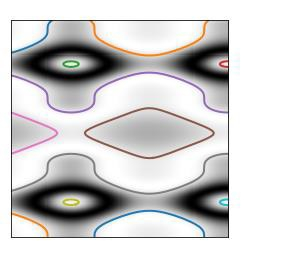# How to find contours of an image using scikit-learn in Python?

Scikit-learn, commonly known as sklearn is a library in Python that is used for the purpose of implementing machine learning algorithms. It is an open-source library hence it can be used free of cost. This library is built on Numpy, SciPy and Matplotlib libraries.

The method of ‘marching squares’ is used to find the contours in an image. The function ‘find_contours’ present in the ‘measure’ class of ‘skimage’ library is used. In this, the values present in the array are interpolated in a linear manner.

This way, the precision of the contours in the output image would be much better. If the contours in the image intersect, the contours are open, else they are closed.

Let us understand how to find contours in an image using scikit-learn library −

## Example

import numpy as np
import matplotlib.pyplot as plt
from skimage import measure
x, y = np.ogrid[-6.7:np.pi:215j, -1.2:np.pi:215j]
r = np.sin(np.exp((np.sin(x)**3 + np.cos(y)**2)))
contours = measure.find_contours(r, 0.8)
fig, ax = plt.subplots()
ax.imshow(r, cmap=plt.cm.gray)
for contour in contours:
ax.plot(contour[:, 1], contour[:, 0], linewidth=2)
ax.axis('Image')
ax.set_xticks([])
ax.set_yticks([])
plt.show()

## Output## Explanation

• The required packages are imported into the environment.

• Data is generated with the help of NumPy package.

• The function ‘find_contours’ is used to determine the contours of the image.

• The ‘subplot’ function is used to show the original image and the image with contours on the console.